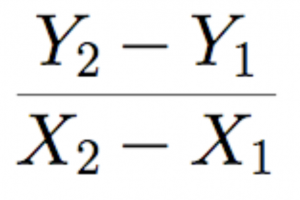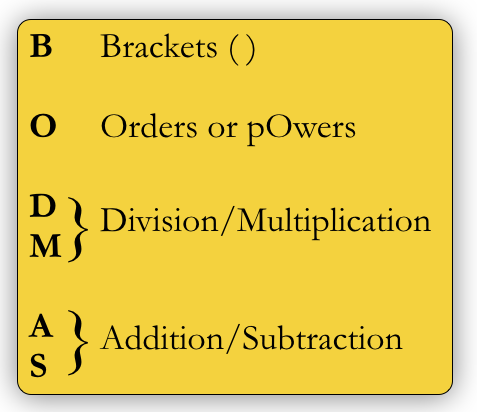# Expressions

Expressions are a “mathematical phrase”, basically a sentence in a language that evaluates to something, usually, but not always, a number.

For example, 1 + 1  is an expression. The + is on operator, because it performs some sort of mathematical operation, while the numbers are operands, that is, the things being operated on.

Similarly, in this expression (the formula for the slope of a line between two points (x1,y1) and (x2,y2)

.we have operands (the x and y values) and operators, such as the  signs and the division. Notice that even though we can’t evaluate this at the moment because we’re missing values for the x1, x2, y1 and y2, this is still an expression.

Evaluating Expressions

When we have all the values needed to evaluate an expression, we need to know the order in which to do it. For example:

3 * 2 + 1 = ?

We could get either

6 + 1 = 7

or

3 * 3 = 9

depending on the order in which we do this. To ensure that everyone gets the same results, there are standard rules of precedence for standard operators:

1. ()
2. *, /
3. +, –

If there’s a tie, when we go from left to right. In that case, it doesn’t matter what the order is, because these operators are associative.

A handy way to remember the order is BODMAS:The order of precedence of arithmetic operators

Although the rules are very simple, evaluating the expressions isn’t trivial once they grow in complexity. Try these exercises below. You can try as many as you want as the book can generate ones for you.

So far we’ve been using infix representation, which is where the operator comes between the operands, but there are other types too. These include prefix, where the operator comes first, for example with things like Sin and Cos, so we end up combinations of representations like this: $$\cos (\sqrt {a + b} )$$.

Now things are starting get hairy, and there are more representations too, such as super-fix, in which the operators goes above the operand ($$x^y$$) and sub-fix where the operator goes underneath the operand, ($$\log_xY or \frac{2}{5}$$).

This means we have to understand each representation and be able to recognise it, but also means that we can pack a lot more information into the same space. Try the following exercises that have a mix of representations:

To make expressions reusable we often have variables that require values, such as in this expression, which is used to derive the roots for quadratic equations (equations of the form $$ax^2 + bx + c = 0$$):

$$\frac{-b \pm \sqrt{b^2-4ac\;\;}}{2a}$$

This has all four representations mixed together which, although convenient for us because we know them all, makes a bunch of assumptions that could lead to confusion if this is being read by someone who is seeing it for the first time. Even worse, if this was being read by a computer that has no understanding of the connection between the expression and the equation, there are a number of areas where confusion could arise. For example:

• Does the length of the square root have to be that long? What if this was written by hand and it was too short?
• The length of the line over the 2a has to be just right. If it is too short, then maybe the -b part won’t be over it and we’ll change the answer.
• For that matter, how is the computer supposed to know that 2a is actually (2 * a)? What’s the big secret?
• Finally, how does it know that ac is (a * c) and not a variable called ac?

These aren’t really problems for us as we can see the whole expression and know how is connected to $$ax^2 + bx + c = 0$$, so we’re much smarter than any computer, but our job here is communicate with the computer. We need to explain everything to it as clearly and unambiguously as possible.Syntax and Semantics

These are two key definitions for success at λ calculus, Computer Science and, let’s face it, life itself! Syntax is concerned with what an expression or program looks like. It’s concerned with where you put semi colons and inverted commas, basically making sure that the program follows all the rules so that it can be compiled or interpreted. What’s far more interesting are semantics, because that’s concerned with what an expression or program does. Truly skilled Computer Scientists know many languages and will choose the most appropriate one for whatever task they are confronted with, often after having performed their design. This is what we want to be able to do, and to do that we want to separate semantics from syntax.

Let’s get back to our expression. How would we type this into a computer? First let’s get it spread out on a single line:

$$(-b \pm \sqrt{b^2-4 * a * c})(2 * a)$$

We still have a problem with the square root sign. The difficulty is that it has variable scope, meaning that the area in which it takes effect varies depending on how long the line is. However, we could just everything in brackets and raise it to the power of $$\frac{1}{2}$$ like this: $$(b^2-4 * a * c)^\frac{1}{2}$$. This looks better and now we can type it into the computer, but what if we want to evaluate it? Does the computer have to read the whole thing in before it knows what to do? Consider if we wanted to evaluate $$(b^2 – 4ac)$$. We read the b, but don’t know what to do until we read the squared sign. At that point we can actually calculate the value, but next we read the minus sign. What happens now? Do we subtract the next thing? Or do we have to evaluate the next part of the expression first?

This is known as the prefix problem. Basically, we don’t know what to do with an incoming string of characters (or numbers or parts of an expression) until we have all of it. We see this problem all the time when sending texts on our phones. The instant we type a letter, the phone is scrambling to figure out what word we want to type. It does this through a combination of words that we typically use, words that we’ve already typed and the letters it has just seen, but the number of possible words is huge. Imagine I open up a new text message and type F. There are a lot of words that start with F, right? Use the slider to add letters to it and see how far you have to go before you guess the word.

How does all this relate to expressions and computers? Let’s take a look at the notion of Locality of Reference in the next section and we’ll start to see how this will all come together.

Back to index.